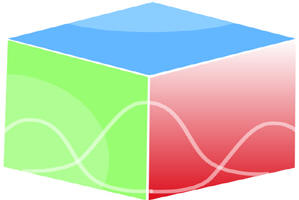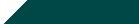``Home| Journals | Statistics Online Expert | About Us | Contact UsUntitled Document

[

Estimation of the Parameter in the Size-Biased Inverse Maxwell Distribution

Kusum Lata Singh1*, R.S. Srivastava2**

1, 2 Department of Mathematics and Statistics, DDU Gorakhpur University, Gorakhpur, INDIA.

Research Article

Abstract: In this paper, the estimation problem of size-biased inverse Maxwell distribution is considered. The parameters are estimated by the method of maximum likelihood estimation and moment equation estimation. The properties of size-biased inverse Maxwell are discussed and its survival and hazard functions are obtaining. These are plotted and their properties have been investigated and test for SBIMD is suggested.

Keywords: SBIMD, Moments, Hazard function, Survival function, Moment method, MLE etc.

Introduction

The concept of weighted distribution can be traced to Fisher in his paper “The study of effect of methods of ascertainment upon estimation of frequencies” in 1934; while the terminology of length-biased sampling was introduced by Cox 1962 (see Patil 2002). These two concepts find various applications in biomedical area such as family history and disease, survival and intermediate events and latency period of AIDS due to blood transfusion (Gupta and Akman 1995). The study of human families and wildlife populations was the subject of an article developed by Patil and Rao (1978). Patil, et al. (1986) presented a list of the most common forms of the weight function useful in scientific and statistical literature as well as some basic theorems for weighted and size-biased distributions;

Let Y is a random variable having the Inverse Maxwell Distribution (Singh, Kusum lata .and Srivastava, R.S.,(2014)” with pdf given by,

f (y; θ) =  . y>0, θ>0                                (1.1)

Where θ is a scale parameter, for the IMD, the raw moment are given by

E(Xr) =  =

=  Ґ()                                                             (1.2)

The mean, variance and harmonic mean are obtained as

Mean =  =                                                                 (1.3)

Variance = =                                                                    (1.4)

Harmonic Mean =                                                                     (1.5)

In this paper we have obtained the p.d.f. of size-biased inverse Maxwell distribution and obtained its mean, median, harmonic mean and mode The parameters is estimated by the method of maximum likelihood estimation and moment equation estimation. The properties of SBIMD are discussed and its survival and hazard functions are obtaining. These are plotted and their properties have been investigated and test for size-biasedness is conducted. Let Y be a random variable having the size-biased inverse Maxwell distribution, its pdf comes out to be,

g(y;θ) =

=  y>0                                                                  (1.6)

Here we get,

Figure 1: pdf of size-biased inverse Maxwell distribution

In the graphs of Size-Biased Inverse Maxwell distribution for various values of parameter θ is given in above figure. The figure shows that the density function can take different shapes for different values of the parameter. The curve is positively skewed and has a long right tail.

Moments

The sized-biased inverse Maxwell distribution, the rth raw moments are given by

E(Xr) =  =

=  Ґ(1-)                                                                              (1.7)

The rth raw moment exist except for r = 2 and therefore no rth central moments are exists for r ≥ 2.

Various Measures of Size - Biased Inverse Maxwell Distribution

Mean

Me =                                                              (1.8)

Solving the equation (1.8), we get

Me = =1.772453851                                                (1.9)

Median

M = =                                                         (1.10)

Solving the equation (1.10), we get

M =  =1.82615729                                         (1.11)

Mode

Mo =  = 0                                                         (1.12)

Solving the equation (1.12), we get

Mo = = 0.81649658                                             (1.13)

Harmonic Mean

=                                                                (1.14)

Solving the equation (1.14), we get

H =  = 1.128379167                                              (1.15)

Table 1

 Theta Mean Median Mode Harmonic Mean 0.09 5.90818 6.087191 2.721655 3.76126389 0.1 5.604991 5.774816 2.581989 3.568248232 0.2 3.963327 4.083412 1.825742 2.523132522 0.5 2.506628 2.582576 1.154701 1.595769122 1 1.772454 1.826157 0.816497 1.128379167 1.5 1.447203 1.491051 0.666667 0.921317732 2 1.253314 1.291288 0.57735 0.797884561 2.5 1.120998 1.154963 0.516398 0.713649646 3 1.023327 1.054332 0.471405 0.651470016 3.5 0.947416 0.976122 0.436436 0.603144035 4 0.886227 0.913079 0.408248 0.564189584 4.5 0.835543 0.860859 0.3849 0.531923041 5 0.792665 0.816682 0.365148 0.504626504 5.5 0.755777 0.778676 0.348155 0.481142493 6 0.723601 0.745526 0.333333 0.460658866 6.5 0.695214 0.716278 0.320256 0.442586722 7 0.669925 0.690223 0.308607 0.426487237 7.5 0.647209 0.666818 0.298142 0.412025815 8 0.626657 0.645644 0.288675 0.39894228 8.5 0.607947 0.626367 0.280056 0.387030861 9 0.590818 0.608719 0.272166 0.376126389 9.5 0.57506 0.592484 0.264906 0.366094544 10 0.560499 0.577482 0.258199 0.356824823 10.5 0.546991 0.563564 0.251976 0.348225371 11 0.534415 0.550607 0.246183 0.34021912 11.5 0.522668 0.538504 0.240772 0.332740863 12 0.511663 0.527166 0.235702 0.325735008 12.5 0.501326 0.516515 0.23094 0.319153824 13 0.49159 0.506485 0.226455 0.312956073 13.5 0.482401 0.497017 0.222222 0.307105911 14 0.473708 0.488061 0.218218 0.301572018 14.5 0.465469 0.479572 0.214423 0.296326887 15 0.457646 0.471512 0.210819 0.291346248

Cumulative distribution function

The cumulative distribution function (CDF), or just distribution function, describes the probability that a real-valued random variable Y with a given probability distribution will be found to have a value less than or equal to y. In the case of a continuous distribution, it gives the area under the probability density function from minus infinity to y. Cumulative distribution functions are also used to specify the distribution of multivariate random variables. Now, consider the cumulative distribution function of size biased Inverse Maxwell distribution

G (t) =                                                           (2.1)

=dy                                                            (2.2)

Putting  ; = du

Limits y = 0 ; u = ; y=t ; u =

G(t) = du =                                                 (2.3)

Figure 2: cdf of Size-Biased Inverse Maxwell distribution

Survival function

The probability that an individual survives longer than t, is called the survival function and denoted by S (t).Let T denote the survival time i.e.

S (t) = P (an individual survives longer than time t)

=1- P ( an individual fails before or at time t)

S (t) =1-G (t) v                                                                      (3.1)

=1-                                                                                 (3.2)

For a survival function, the t value on the graph starts at one and monotonically decreases to zero. The survival function should be compared to the cumulative distribution function.

As t ranges from 0 to , the survival function has the following properties

It is non-increasing

2. At time t = 0, S(t) = 1. In other words, the probability of surviving past time 0 is 1.

3. At time t = , S(t) = S() = 0. As time goes to infinity, the survival curve goes to 0.

Figure 3: the survival function of size-biased inverse Maxwell distribution for various values of θ

Hazard function

The hazard function of survival time T gives the conditional failure rate, it is defined as the probability of failure during a very small time interval, assuming that the individual has survived to the beginning of the interval, or as the limit of the probability that an individual fails in a very short interval, t+∆t, given that the individual has survived to time t:

λ(t)=

λ (t) =                                                                         (4.1)

λ (t) =                                                                              (4.2)

=                                                                  (4.3)

The SBIMD’s hazard function has a unique behavior; it increases initially, then decreases and eventually approaches zero. This means that items with a SBIMD have a higher chance of failing as they age for some period of time, but after survival to a specific age, the probability of failure decreases as time increases. The size- biased inverse Maxwell distribution’s hazard function is shown in the figure below.

Figure 4: Hazard function of Size-Biased Inverse Maxwell distribution

Estimation of parameter

Method of moment equation estimation

Let Y1,Y2,Y3………Yn be an independent sized biased sample ,then the method of moments equation estimations are obtained by setting the moments E(Y)equal to the corresponding sample moments that is

= E(Y) =                                                                           (5.1)

=

Squaring both sides, we get

=  =  ;                                                                      (5.2)

Maximum likelihood estimator

Again, the estimations of the parameters of Size-Biased Inverse Maxwell distribution are discussed by the method of MLE using Equation (2).the maximum log likelihood function of SBIMD may be expressed as

L =  ;                                                                 (5.3)

L =

=

Taking log on the both sides, we have

Log L=n [log 2- log θ] +log -

(5.4)

Differentiation above eqn w. r. t. θ and setting the result equal to zero, we have

=  +                                        (5.5)

Put  = 0

=  =                           (5.6)

As the MLE of θ

Test for size-biased inverse Maxwell distribution

Let Y1, Y2,……,Yn be random samples can be drawn from Inverse Maxwell distribution or Size-biased Inverse Maxwell distribution. We test the hypothesis H0:f (y) = f (y;θ) against H1:f(y) = fs (y;θ) To test whether the random sample of size n comes from the Inverse Maxwell distribution or size-biased Inverse Maxwell distribution .the following test statistics is used

∆ =  =                                                        (6.1)

=                                                  (6.2)

∆ =                                                     (6.3)

We reject the null hypothesis

> k                                                    (6.4)

Equalivalently, we reject the null hypothesis where

∆* =                                                      (6.5)

Where k* = k > 0

For a large sample size of n, 2log∆ is distributed with one degree of freedom. Thus, the p-value is obtained from the chi-square distribution.

Conclusion

In this paper, the SBIMD from of the weighted IMD named as new class of SBIMD. A SBIMD is a particular case of weighted inverse Maxwell distribution, taking the weights as the variate values has been defined. The p.d.f., c.d.f., survival and hazard function has been plotted. The estimates of the parameters of SBIMD are obtained by employing the method of moment equation

=

and MLE of θ is

=, As the MLE of θ

Also, a test for detecting the Size-biasedness is suggested.

References

1. Cox, D. F., Description and measurement of rates of early mortality in the pig. Iowa State Univ. Agr. Expt. Sta. Res. Bull. 500, 1962.
2. Gupta, R.C. and Akman, H.O. On the reliability studies of a weighted inverse Gaussian model. Journal of Statistical Planning and Inference 48, 69-83. 1995.
3. Fisher,R.A., “The study of effect of methods of ascertainment upon estimation of frequency “,.1934.
4. Patill, G.P. and Rao, C.R. Weighted distributions and size-biased sampling with application to wildlife populations and human families. Biometrics, 34,179- 189. 1978.
5. Patill, G.P. and Rao, C.R. and Ratnaparkhi, M.V. On discrete weighted distributions and their use in model choice for observed data. . Commun. Statist. –Theory Meth, 15(3), 907-918. 1986.
6. Patill, G.P., Weighted distributions. Encyclopedia of Environmetics, Johon Wiley and Sons. Vol.4, 2369- 2377, 2002.
7. Singh, Kusum lata and Srivastava, R.S.,” INVERSE MAXWELL DISTRIBUTION AS A SURVIVAL MODAL,GENESIS AND PARAMETER ESTIMATION”(accepted)Research Journal of Mathematical and Statistical Sciences, Vol 2 issue 7, 2014.